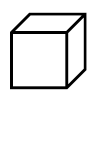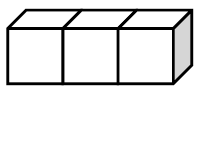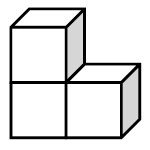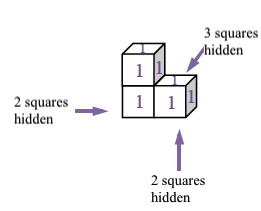### Home > CCAA > Chapter 9 Unit 8 > Lesson CC2: 9.2.1 > Problem9-56

9-56.

Find the volume and surface area of each shape below. Assume that the edge of each cube measures $1$".

1.Each of these objects are three-dimensional and have length, width, and depth.

Volume is the number of $1\text{-inch}$ cubes needed to fill a three dimensional object.

The surface area of an object is the total area of all its surfaces.

1.See part (a).

1.Including the hidden faces, there are $14$ total squares, each with area 1 sq in. There are $3$ cubes so the volume is $3$ in³.